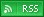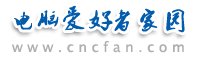电脑爱好者，提供IT资讯信息及各类编程知识文章介绍，欢迎大家来本站学习电脑知识。 最近更新 | 联系我们站内搜索：

# VBScript 与窗体

<HTML>
<SCRIPT LANGUAGE="VBScript">
<!--
Sub Submit_OnClick
Dim TheForm
Set TheForm = Document.ValidForm
If IsNumeric(TheForm.Text1.Value) Then
If TheForm.Text1.Value < 1 Or TheForm.Text1.Value > 10 Then
MsgBox "请输入一个 1 到 10 之间的数字。"
Else
MsgBox "谢谢。"
End If
Else
MsgBox "请输入一个数字。"
End If
End Sub
-->
</SCRIPT>
<BODY>
<H3>简单验证</H3><HR>
<FORM NAME="ValidForm">

<INPUT NAME="Text1" TYPE="TEXT" SIZE="2">
<INPUT NAME="Submit" TYPE="BUTTON" VALUE="提交">
</FORM>
</BODY>
</HTML>

A = Text1.Value + Text2.Value ' A 为“12”
A = CDbl(Text1.Value) + Text2.Value ' A 为 3

<SCRIPT LANGUAGE="VBScript">
<!--
Sub Submit_OnClick
Dim TheForm
Set TheForm = Document.ValidForm
If IsNumeric(TheForm.Text1.Value) Then
If TheForm.Text1.Value < 1 Or TheForm.Text1.Value > 10 Then
MsgBox "请输入一个 1 到 10 之间的数字。"
Else
MsgBox "谢谢。"
TheForm.Submit ' 数据输入正确，传递到服务器。
End If
Else
MsgBox "请输入一个数字。"
End If
End Sub
-->
</SCRIPT>

 Vbscript热门文章排行 网站赞助商